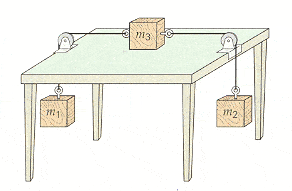# A body on a table with friction.

• dttah

#### dttah

Hello, I'd like to know if this is correct ... thank you.

## Homework Statement

You are given that the coefficient of kinetic friction between the block and the table in the attachment is 0.560, and m1 = 0.135 kg and m2 = 0.260 kg. (a) What should m3 be if the system is to move with a constant speed? (Assume ideal conditions for the string and pulleys.)## The Attempt at a Solution

Start by writing down all of the forces involved in m1 and m2...

$\Sigma F_{m1} = T_1 - m_1g = m_1a$
$\Sigma F_{m2} = T_2 - m_2g = m_2a$
$\Sigma F_{m3} = T_2 - T_1 - \mu m_3g = 0$
I write = 0 because constant speed means no acceleration... so no acceleration means that F = ma => F = 0.

Now I have to solve this thingy, solve for T1 And T2 in the first two and stick it in the third.. getting..
$T_1 = m_1g + m_1a$
$T_2 = m_2g + m_2a$
$m_2g + m_2a - m_1g - m_1a - \mu m_3g = 0$

Okie dokie, now we are able to solve for m3...
$m_3 = \frac{m_2g + m_2a - m_1g - m_1a}{\mu g} \rightarrow \frac{m_2g - m_1g}{\mu g}$

$\frac{1,225}{5.488} = 0.2232$

$T_1 = m_1g + m_1a$
$T_2 = m_2g + m_2a$
Since a = 0, you should immediately write T1 = m1g and T2 = m2g.

$m_3 = \frac{m_2g + m_2a - m_1g - m_1a}{\mu g} \rightarrow \frac{m_2g - m_1g}{\mu g}$
Good, but cancel those g's. The more you can cancel and simplify before reaching for the calculator the better.

The result looks good.

Note that certain shortcuts present themselves when you are given that velocity is constant; Since there's no acceleration your formulas for the tensions can simplify accordingly. No need to carry any acceleration terms after that point. The net horizontal force on m3 is then just the difference in the tensions.

Yeah, I noticed I had to write a = 0 just afterwards and I was too lazy to correct all the latex. Good point about the g's, will do next time. Thank you! ;)